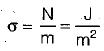Courses

# Test: Properties of Fluids - 2

## 10 Questions MCQ Test Topicwise Question Bank for Mechanical Engineering | Test: Properties of Fluids - 2

Description
This mock test of Test: Properties of Fluids - 2 for Mechanical Engineering helps you for every Mechanical Engineering entrance exam. This contains 10 Multiple Choice Questions for Mechanical Engineering Test: Properties of Fluids - 2 (mcq) to study with solutions a complete question bank. The solved questions answers in this Test: Properties of Fluids - 2 quiz give you a good mix of easy questions and tough questions. Mechanical Engineering students definitely take this Test: Properties of Fluids - 2 exercise for a better result in the exam. You can find other Test: Properties of Fluids - 2 extra questions, long questions & short questions for Mechanical Engineering on EduRev as well by searching above.
QUESTION: 1

Solution:
QUESTION: 2

Solution:
QUESTION: 3

### When subjected to shear force, a fluid

Solution:
QUESTION: 4

An Ideal Fluid

Solution:

Ideal Fluid: A fluid, which is incompressible and is having no viscosity is known as ideai fluid. Ideal fluid is only an imaginary fluid as all the fluids, which exists have some viscosity.

QUESTION: 5

Which one of the following is defined as force per unit length?

Solution:

Surface tension is defined as line force per unit length.

QUESTION: 6

Newton’s law of viscosity depends upon the

Solution:

According to Newtons law of viscosity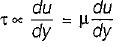QUESTION: 7

The fluid which obey’s the Newton’s Saw of viscosity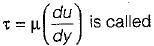Solution:

The fluid which obeys the Newton’s law of viscosity is known as Newtonion fluid e.g.: Air, water, Kerosene.

QUESTION: 8

The General relationship between shear stress (τ) and velocity Gradient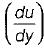for a fluid can be written as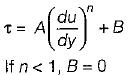Then the fluid known as

Solution:

In the expression: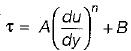If n < 1, B = 0
then it is known as pseudo plastic

QUESTION: 9

Surface tension is due to

Solution:

Surface tension a line force caused by cohesion of fluid particle at the surface, the property of cohesion enables a liquid to resist tensile stress.

QUESTION: 10

Which one of the following is correct dimension of surface tension?

Solution: